# A Comparative Study on Sorting Algorithms

“In computer science, a sorting algorithm is an algorithm that puts elements of a list in a certain order. The most frequently used orders are numerical order and lexicographical order.”

# Sorting Algorithms

The sorting techniques can be divided into two categories:
Internal Sort : In internal sorting, all the data to sort is stored in memory at all times while sorting is in progress. e.g : bubble sort, quick sort, insertion sort, selection sort , etc.
External Sort : In external sorting data is stored outside memory (like on disk) and only loaded into memory in small chunks. External sorting is usually applied in cases when data can’t fit into memory entirely. e.g : merge sort , heap sort , etc.

# Bubble Sort

Step 1: Consider the set of elements which can be of any data for the given lists. 
Step 2: Swap first two data for the given lists adjacently using one by one pairs. 
Step 3: Repeat the process for all the items or data in the given list. 
Step 4: Return result for the same.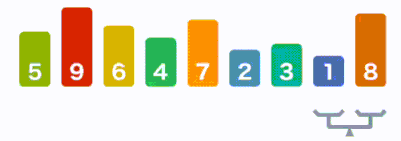Bubble Sort [Source : Google]

Advantages:
◾ It is one of the popular and easily implemented algorithms.
◾ Without using the extra storage, the items can be swapped easily.
◾ Requires minimum space for given lists.

Disadvantages:
◾ Requirement of N-squared number steps for sorting items.
◾ Does not deal with large number of items for the given lists.
◾ Cannot be used for real time applications.

# Selection Sort

Step 1: First finds the smallest element for the given lists of item or data. 
Step 2: Replaces that item or data in the first position. 
Step 3: Next, again finds the smallest element among the lists. 
Step 4: Get replaced in second position. 
Step 5: Same procedure is followed unless the elements are sorted. 
Step 6: Returns result.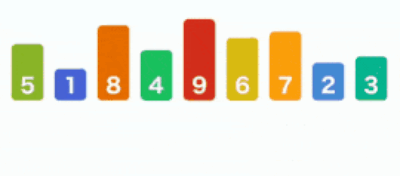Selection Sort [Source : Google]

Advantages:
◾ Before sorting the given lists, the ordering of data can be initialized.
◾ This works even for the smaller lists of items or data.
◾ Need no extra storage for the original lists of items.

Disadvantages:
◾ For the large set of items or data this sorting results in poor efficiency.
◾ Requires an N-squared number steps for sorting items for the given lists.
◾ Compared to selection sort, the quick sort is most efficient.

# Quick Sort

Step 1: Pick the element from the given list and use as a pivot.
Step 2: Partition these elements into two sub-lists.
Elements less than pivot.
Elements greater than pivot.
Step 3: Quick sort two sub-lists.
Step 4: Repeat the process until the entire lists of item are sorted.
Step 5: Return result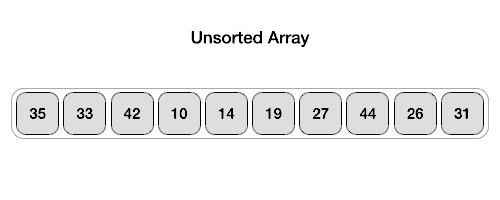Quick Sort [Source : Google]

Advantages:
◾ This is one of the best sorting algorithms.
◾ It can handle large items for the given lists.
◾ Does not require extra storage.

Disadvantages:
◾ The worst-case performance is same as bubble, selection and insertion sort.
◾ In case the given lists are already sorted, then bubble sort is more efficient compared to quick sort.
◾ For sorting the integers of given items, radix sort plays an important role as compared to quick sort.

# Insertion Sort

Step 1: If the first element is already sorted, Return 1;
Step 2: Take the next element
Step 3: Compare the element with all other elements in sorted sub-list
Step 4: Move all the elements in the sorted sub-list which is bigger than the Value to be sorted
Step 5: Insert the value
Step 6: Repeat until list is sorted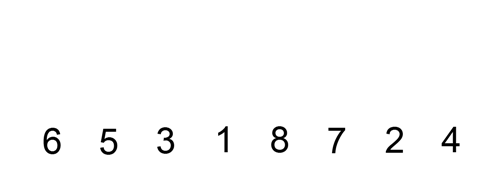Insertion Sort [Source : Google]

Advantages:
◾ Simple implementation
◾ Efficient for small data sets
◾ It is stable

Disadvantages:
◾ Less efficient for the list containing more elements
◾ It needs large number of element shifts

# Merge Sort

Step 1: If the first element is already sorted, return 1.
Step 2: The list is divided recursively into two equal parts until it cannot be divided.
Step 3: Combine the smaller list of data items into the new list which has sorted elements.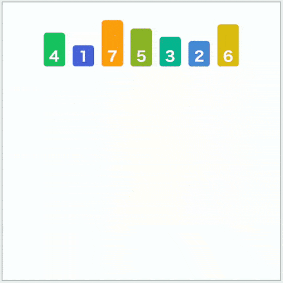Merge Sort [Source : Google]

Advantages:
◾ Merge sort can be useful to files of any size.
◾ It is fast and stable

Disadvantages:
◾ Merge Sort takes more space when compared to other sorts.
◾ Merge sort is less efficient than other sort

# Heap Sort

Step 1: Build a max heap from the input data.
Step 2: At this point, the largest item is stored at the root of the heap. Replace it with the last item of the heap followed by reducing the size of heap by 1. Finally, heapify the root of tree.
Step 3: Repeat above steps while size of heap is greater than 1.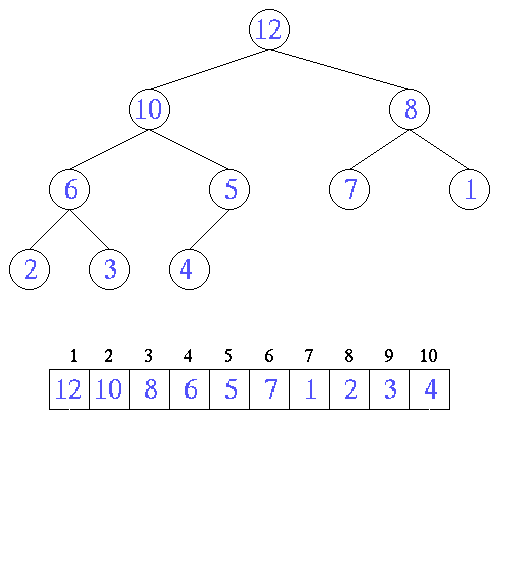Heap Sort [Source : Google]

Advantages:
◾ Because of its efficiency, this algorithm is widely used.
◾ As it is an in-place sorting algorithm its memory usage is nominal.

Disadvantages:
◾ More space is required for sorting
◾ Quick sort is more efficient when compared to Heap in most of cases

# Performance in Average Case Between Sorting Algorithms

Implementation of Selection sort, Quick sort, Insertion sort , Merge sort and Bubble sort algorithms using C++ programming language is done and execution time of all programs is measured with the same input data using the same computer. The built-in function (clock ()) in C++ is used to get the elapsed time of the implementing algorithms, execution time of a program is measured in milliseconds. The performances conventional sort algorithms are comparatively tested under average cases by using random test data from size 10000 to 30000.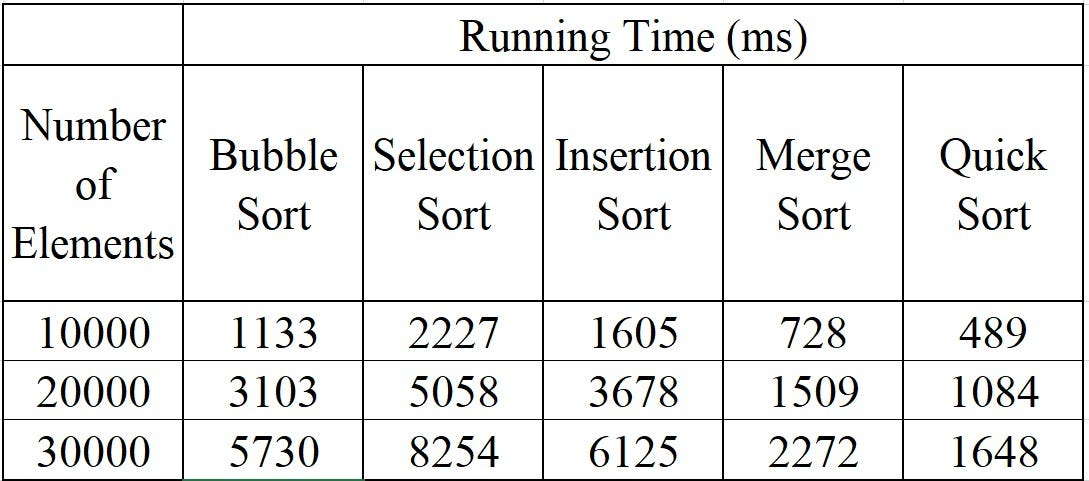Comparative Performances of Sorting Algorithms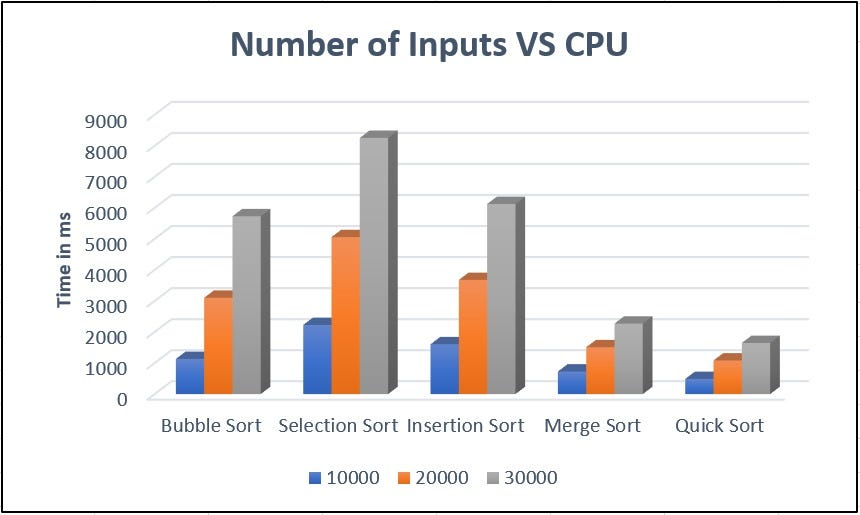Plot of Number of Input vs CPU.

# Complexity Comparison between Typical sorting algorithms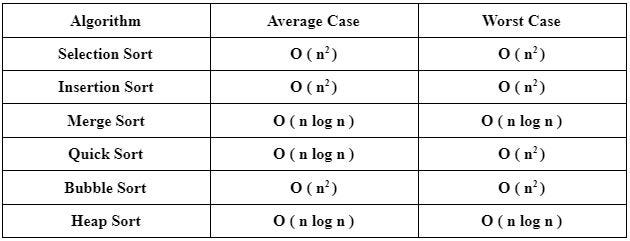Time Complexity of Typical Sorting Algorithms.

# Conclusion

Comparative study of sorting algorithms like selection sort, Insertion sort, merge sort, quick sort, heap sort and bubble sort is done. Performance of these algorithms is analyzed by taking same number of elements/inputs (10000,20000, 30000). For small number of inputs, the performance for the six techniques is almost nearest, but for the large number of inputs, Quick sort is the fastest and the selection sort the slowest. In average and worst case, the time complexity with selection, Insertion and bubble sort is nearly same. This research is initial step for future work, in the future we will improve our research on various such algorithms to optimize software’s in searching method and retrieve data.